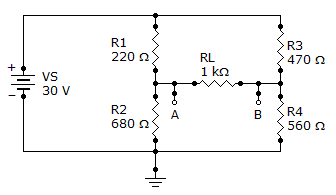# Electrical Engineering - Circuit Theorems and Conversions

### Exercise :: Circuit Theorems and Conversions - General Questions

11.

What is the Thevenin equivalent (VTH and RTH) for the circuit given?A. 6.4 V, 422B. 6.4 V, 560C. 6.4 V, 680D. 30 V, 422Explanation:

No answer description available for this question. Let us discuss.

12.

Referring to the given circuit, the voltage and current for the load resistor, RL, isA. 450 mV, 4.5 mA B. 4.50 V, 45 mA C. 4.50 V, 4.5 mA D. 450 mV, 45 mA

Explanation:

No answer description available for this question. Let us discuss.

13.

Find the current in R2 of the given circuit, using the superposition theorem.A. 16.7 mA B. 33.3 mA C. 50 mA D. 16.6 mA

Explanation:

No answer description available for this question. Let us discuss.

14.

A 470RL is connected across a voltage source, VS, of 120 V. The source's internal resistance, RS, is 12. What is the output voltage across the load?

 A. 120 V B. 0 V C. 117 V D. 12 V

Explanation:

No answer description available for this question. Let us discuss.

15.

A 2RL is connected across a voltage source, VS, of 110 V. The source's internal resistance is 24. What is the output voltage across the load?

 A. 8.5 V B. 85 V C. 0 V D. 110 V